# Gradient descent with constant learning rate for a logistic log-loss function of one variable

## Setup

This page includes a detailed discussion of gradient descent with constant learning rate for the logistic log-loss function of one variable.

### Function

Explicitly, the function is: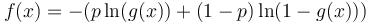$f(x) = -(p \ln (g(x)) + (1 - p) \ln(1 - g(x)))$

where$g$ is the logistic function and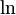$\ln$ denotes the natural logarithm. Explicitly,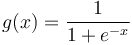$g(x) = \frac{1}{1 + e^{-x}}$.

Note that$1 - g(x) = g(-x)$, so the above can be written as:$f(x) = -(p \ln (g(x)) + (1 - p) \ln (g(-x)))$$p \in (0,1)$ (we avoid extremes of 0 and 1 because in the extreme case the optimum is at infinity).

More explicitly,$f$ is the function:$f(x) = p \ln(1 + e^{-x}) + (1 - p) \ln (1 + e^x)$

The optimal value that we want to converge to is: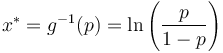$x^* = g^{-1}(p) = \ln \left(\frac{p}{1 - p} \right)$

### Learning algorithm

Suppose$\alpha$ is a positive real number. The gradient descent with constant learning rate$\alpha$ is an iterative algorithm that aims to find the point of local minimum for$f$. The algorithm starts with a guess$x^{(0)}$ and updates according to the rule: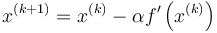$x^{(k+1)} = x^{(k)} - \alpha f'\left(x^{(k)}\right)$

Concretely, this is:$x^{(k+1)} = x^{(k)} - \alpha\left( g\left(x^{(k)}\right) - p \right)$

Note that we use parenthesized superscripts to denote the iterates, and these should not be confused with exponents. The reason for using superscripts instead of subscripts is to keep notation consistent with the case of functions of multiple variables, where subscripts are used for coordinates and superscripts for iterates.

## Convergence properties

To guarantee good global convergence, we need to choose a learning rate that takes into account the global upper bound on the second derivative. We have that the second derivative is: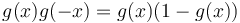$g(x)g(-x) = g(x)(1 - g(x))$

This is maximized at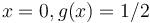$x = 0, g(x) = 1/2$, with value$1/4$. Therefore, the bound$B$ on the second derivative, as discussed on the page gradient descent with constant learning rate for a convex function of one variable, is$1/4$, and so, what we are guaranteed is that any learning rate in in the interval$(0, 2/B) = (0,8)$ will work globally, and a learning rate in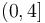$(0,4]$ will work globally with monotone convergence towards the optimum.

For local convergence, it suffices to choose a learning rate that is in the interval: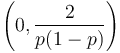$\left(0, \frac{2}{p(1 - p)}\right)$

with the best convergence (superlinear convergence) occurring if the learning rate chosen is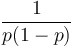$\frac{1}{p(1 - p)}$.

Now, it turns out that even using a learning rate in this interval will still guarantee global convergence. But the first few steps could be really bad, so even starting with a point close to the optimum, we may end up moving quite far from the optimum.

### General case

For a learning rate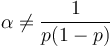$\alpha \ne \frac{1}{p(1 - p)}$, we obtain linear convergence with convergence rate:$|1 - \alpha p(1 - p)|$

Note that, for fixed$\alpha$, this convergence rate goes to 1 (i.e., becomes very slow) as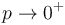$p \to 0^+$ and also as$p \to 1^-$.

Why do we have convergence? As discussed here, if$-f''$ is quasi-convex, the locally optimal learning rate (or anything smaller) also works globally. In this case,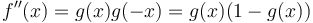$f''(x) = g(x)g(-x) = g(x)(1 - g(x))$ is continuous and attains its unique local maximum at$x = 0$.

In the case that we choose the safe learning rate$\alpha = 4$, we get a convergence rate:$|1 - 4p(1 - p)|$

### Special case of optimal learning rate, probability not equal to 1/2

If we choose$\alpha$ as the optimal learning rate, namely$\frac{1}{p(1 - p)}$ (the reciprocal of the second derivative at the point of minimum), then, as discussed at the page gradient descent with optimal constant learning rate converges quadratically from sufficiently close to a minimum of multiplicity one, we get quadratic convergence, with convergence rate: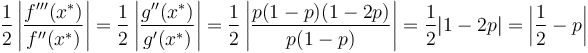$\frac{1}{2} \left| \frac{f'''(x^*)}{f''(x^*)}\right| = \frac{1}{2} \left|\frac{g''(x^*)}{g'(x^*)} \right| = \frac{1}{2} \left| \frac{p(1 - p)(1 - 2p)}{p(1 - p)} \right| = \frac{1}{2}|1 - 2p| = \left|\frac{1}{2} - p \right|$

### Special case of optimal learning rate, probability equal to 1/2

Suppose$p = 1/2$ and$\alpha = 4$. In this case, the above convergence rate works out to 0, suggesting that the convergence is faster than quadratic. This is indeed the case. As described at the page gradient descent with optimal constant learning rate converges quadratically from sufficiently close to a minimum of multiplicity one, we get cubic convergence, with convergence rate: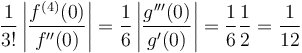$\frac{1}{3!} \left| \frac{f^{(4)}(0)}{f''(0)} \right| = \frac{1}{6} \left| \frac{g'''(0)}{g'(0)} \right| = \frac{1}{6} \frac{1}{2} = \frac{1}{12}$

### Example to illustrate extremely bad initial steps and extremely slow convergence if we use a learning rate based on the second derivative

Suppose we choose$p = g(-100)$, so the optimal value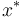$x^*$ is$-100$. Note that$p \approx e^{-100}$. Suppose we choose the optimal learning rate for local convergence. The locally optimal learning rate for convergence is:$\frac{1}{p(1 - p)} \approx e^{100}$

For simplicity, we will take$\alpha = e^{100}$ rather than the exact optimum -- it does not affect anything material, but it simplifies our calculations.

Suppose$x^{(0)} = 0$ (a neutral initial starting point). Then, the first iteration gives: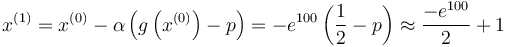$x^{(1)} = x^{(0)} - \alpha\left(g\left(x^{(0)}\right) - p\right) = -e^{100}\left(\frac{1}{2} - p\right) \approx \frac{-e^{100}}{2} + 1$

Note that even though$x^{(0)} = 0$ was quite close to the optimal point$x^* = -100$, the first iterate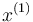$x^{(1)}$ is very very far from it. We did move in the correct direction, but overshot by a very large margin.

However, things will proceed uphill from this point, because in every further iteration, we improve by about 1 unit: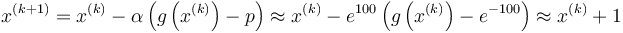$x^{(k+1)} = x^{(k)} - \alpha\left(g\left(x^{(k)}\right) - p \right) \approx x^{(k)} - e^{100} \left(g\left(x^{(k)}\right) - e^{-100}\right) \approx x^{(k)} + 1$

at least when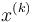$x^{(k)}$ is negative of large magnitude compared with 100. So in each step we come closer to the optimum by about 1 (and more importantly, by at least some number that's slightly less than 1). We will therefore eventually converge. Note, however, that we'd need somewhere in the ballpark of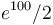$e^{100}/2$ iterations to come approximately as close to the optimum as we were at the outset. Thus, although we technically didn't diverge, for all practical purposes, we did.